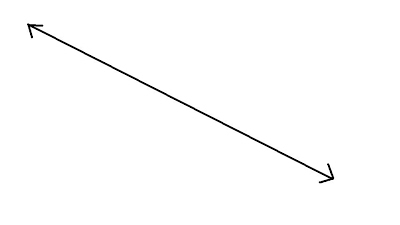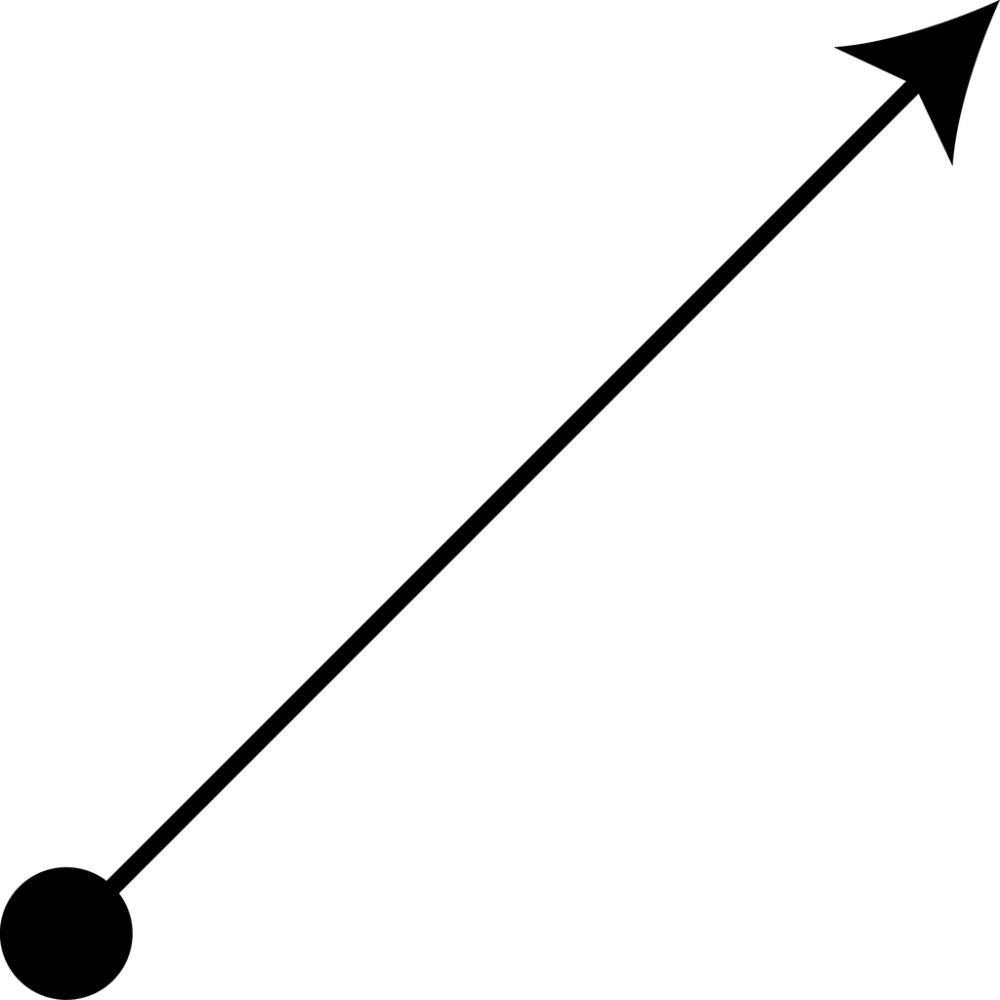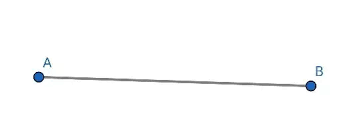4th Grade Multiplication
4th Grade Geometry
4th Grade Division
4th Grade Mental Math
4th Grade Addition and Subtraction
100

4 x 8=

32

100

Draw a line.100

836 divided by 4

209
100

10 x 10=

100

100

492

+204

696

200

72 x 9=

648

200

Draw a ray.200

276 divided by 2?

138

200

200 x 2 =

400

200

497-321

176

300

251 x 5

1,255

300

Draw a line segment.300

321 divided by 2

160 r1

300

20 + 20 + 20 + 20 + 1=

81

300

589

+351

940

400

1,379 x 6 =

8,274
400

Draw a pair of perpendicular lines.400

3,654 divided by 4

913 r 2

400

4 times 15

60

400

8,392

-  492

7,900

500

25 x 11=

275

500

Draw a pair of parallel lines.500

5,745 divided by 9

638 r3

500

1001- 901=

100

500

847

+376

1,223

Click to zoom